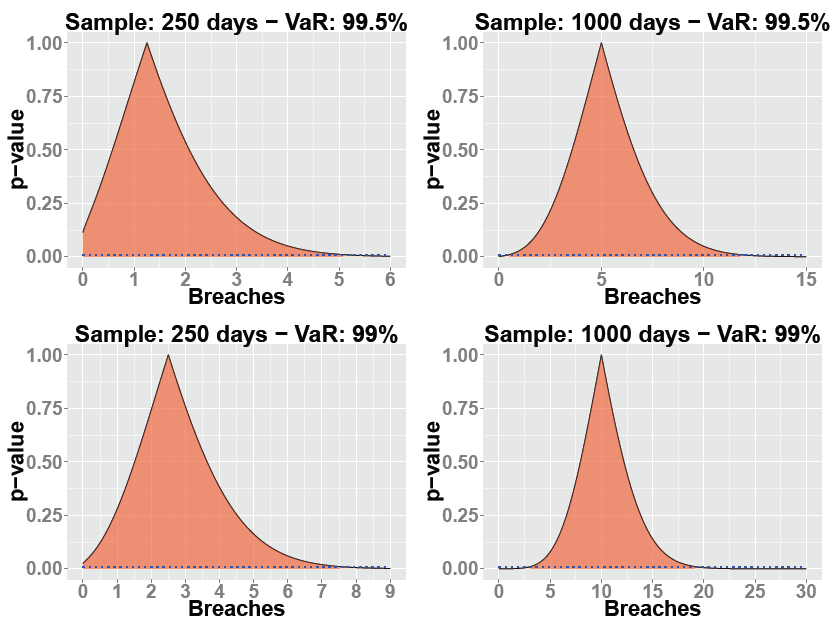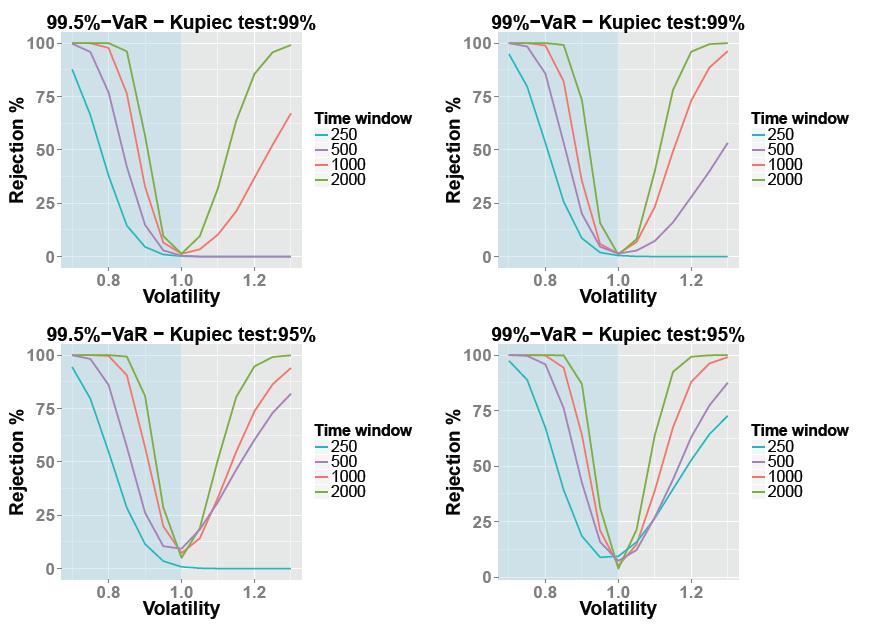# Testing the test: How reliable are risk model backtesting results?

Emmanouil Karimalis, Paul Alexander & Fernando Cerezetti.

All models, including those which model financial risk, are in some sense “wrong” – they aim to “approximate” the real word but cannot possibly recreate it. Consequently, in a world in which risk models are used to calculate and exchange vast sums of capital and margin, the need for reliable tests is of paramount importance. The Kupiec-POF test represents the most widely-used test for assessing the reliability of these risk models (typically Value-at-Risk (VaR) models) – a process known as backtesting. As with all forms of testing, the Kupiec-POF test has a degree of error associated with its use and under certain circumstances these errors may be substantial.

Given the clear systemic risk implications of employing an erroneous model, regulators and risk managers are often concerned with so-called “Type-II” errors (i.e. the non-rejection of an incorrectly specified model). This blog reveals that the distributional nature of the profit and loss (P&L) distribution being modelled can have a significant impact upon the previously known factors driving Type-II errors.

Risk models are not expected to produce reliable and robust risk estimates 100% of the time. Indeed when specifying a model, users build-in expectations around its accuracy often defined by the number of breaches it produces (i.e. occasions whereby the P&L of a portfolio is greater than that predicted by the model). The Kupiec-POF test therefore attempts to assess model performance by comparing the amount of breaches a user would expect a model to produce with the actual amount it does. The test is used by a range of financial institutions and has the following characteristics:

• It involves hypothesis testing – i.e. it has statistical foundations.
• It is two-tailed – i.e. it will fail a model if it has too few or too many breaches for a specific confidence level.

Previous research has highlighted several key relationships between the assumptions and performance of the Kupiec-POF test. In general, the likelihood of observing a Type-II error increases as:

• The size of the backtesting sample falls;
• The confidence interval of the risk model increases;
• The Kupiec-POF test confidence level increases.

Our analysis, based upon a simulation based exercise, validates these relationships but also suggests that the test statistic underlying the Kupiec-POF test and the characteristics of the P&L distribution being modelled can have a sizeable impact on its performance.

The distribution of the Kupiec-POF test statistic

The test statistic of the Kupiec-POF test is assumed to follow a chi-squared distribution. A simple inspection of the test statistic’s non-rejection area, which essentially determines whether or not the model has passed or failed, can prove to be very informative for users of the test.

Figure 1 below shows the test’s relevant p-values on the y-axis and associated VaR model breaches on the x-axis at 99.5% (top panels) and 99% VaR confidence levels (bottom panels) using two sample sizes: 250 and 1000 days’ worth of P&L returns. The red-shaded region denotes the non-rejection area corresponding to a 95% significance level.

Figure 1: Kupiec-POF test’s p-values and non-rejection areasThe charts reveal two key observations:

• As the backtesting sample size increases or the VaR confidence level declines the shape of the non-rejection area becomes “tighter” and more symmetric. This implies that if a user were to do either, the likelihood of experiencing a type II error would be lower.
• Perhaps more interestingly, when the sample size is small (e.g. 250 days) or the VaR confidence level is high (e.g. 99.5%) the shape of the non-rejection area is skewed and bounded to the left. This indicates that the test will struggle to reject a risk model producing zero breaches even if the test has been designed to do so.

These findings suggest that the previously-known limitations of the Kupiec-POF test are, in fact, partly attributable to its statistical foundations.

Interesting results from our simulation-based analysis

One method of assessing the performance of any type of test is to create an artificial environment in which the model’s accuracy is known by its user before the test is undertaken. This provides the user with perfect ex-ante understanding of a model’s performance which also enables sensitivity analysis to be undertaken.

Applying this approach to the Kupiec-POF test, we generate a specific, pre-defined, P&L return distribution and then calibrate a VaR model such that it represents as close to a “perfect” fit as possible. To ensure similarity to real-world financial returns we employ three types of models and P&L distributions: Normal; Standardised Student-t; and a Standardised Skewed Student-t distribution.

In each case, the standard deviation or volatility of the “correct” model, which is set equal to one, is deliberately misspecified using a range of values between 0.7 and 1.3. Here, the model will over-estimate (under-estimate) volatility if its standard deviation is higher (lower) than one, by a maximum of 30%.

Figure 2 below shows Kupiec-POF test rejection rates under a number of different scenarios, with the key assumption that the underlying P&L returns follow a Normal distribution. In each chart, rejection rates and the model’s volatility are displayed on the vertical and horizontal axes respectively. Coloured lines therefore denote the rejection rate for a given rate of volatility using a specific backtesting sample size. The “better” the test, the quicker the rejection rate should rise towards 100% as volatility is misspecified. The top two graphs show the results for the test at the 99% significance level and the bottom graphs show the results for a significance level of 95%. Additionally, graphs on the left and right hand sides represent results for the 99.5% and 99% VaR confidence levels.

Figure 2: Rejection rates for a Normal modelA simple visual inspection of these charts supports our previous expectation that the probability of experiencing a type II error is asymmetric around the correctly specified volatility. In particular, our results suggest that the Kupiec-POF test is in fact less likely to fail a VaR model which over-estimates volatility when compared to one that under-estimates volatility. Interestingly, this phenomenon worsens as the VaR/test confidence interval increases or the sample size is reduced.

Whilst insightful, our analysis has so far relied upon an assumption of normality for the underlying P&L distribution and extensive research has shown that this is unrepresentative for the majority of financial variables (e.g Fama (1963); Fama (1965); Mandelbrot (1963)); which are often skewed and exhibit excess kurtosis (i.e. fatter tails). With this in mind, it’s worth exploring how our initial results might change as we assess the Kupiec-POF test using more representative P&L return distributions.

The figures below therefore show results for the same experiment, now based on a Student-t distribution with three and nine degrees of freedom (red and blue lines respectively) across different sample sizes and model/test confidence intervals. Here, lower degrees of freedom represent a more “fat-tailed” distribution.

Figure 3: Rejection rates for a standardised Student-t modelInterestingly, we can see that as the distribution of the P&L returns becomes more fat-tailed the likelihood of a type II error increases substantially. This is evidenced by analysing the difference between the blue and red lines above. The graphs also reinforce the phenomenon of asymmetry, as explained previously. Again, reducing the backtesting sample size or increasing the VaR/test confidence interval worsens both of these effects.

Extending our analysis to P&L returns which are both fat-tailed and skewed brings us even closer to real-world financial returns. To do so we utilise a standardised skewed Student-t distribution. The figure below presents results for a fat-tailed distribution (three degrees of freedom) with both negative and positive skew – i.e. 0.7 and 1.3 skew parameters, respectively.

Figure 4: Rejection rates for a standardised Skew-t modelAgain, the results support previous analysis regarding backtesting sample sizes, model confidence intervals and the existence of asymmetry. However, it’s also clear that these effects now become much more pronounced when the P&L distribution is more “fat-tailed” and negatively skewed (as the blue lines are always above their red counterparts).

In conclusion, our simulation based analysis has added to existing research on Kupiec-POF test performance by demonstrating that the impact of small backtesting sample sizes and/or high confidence intervals depends crucially upon the characteristics of the underlying P&L distribution.

How can users of the Kupiec-POF test use our findings to improve decision-making?

In practice, many factors affecting Kupiec-POF test performance are difficult to control – e.g. using a large backtesting sample size might not be possible for relatively new contracts or portfolios.  That said, it goes without saying that in order to reduce Type-II errors users should favour longer sample sizes and resist the temptation to utilise low VaR model or test confidence intervals. Our analysis also suggests that one particularly important method of becoming more comfortable with Kupiec-POF test results is the user’s assessment of the underlying P&L distribution’s characteristics (i.e. kurtosis, skewness and tests for normality). By requesting such information, regulators and end-users might ultimately feel less circumspect with a relatively small backtesting sample size if the P&L returns appear normally distributed or alternatively request the use of a much larger backtesting sample size if they are highly skewed and fat-tailed.

Emmanouil Karimalis, Paul Alexander & Fernando Cerezetti work in the Bank’s Risk, Research and CCP Policy Division.

If you want to get in touch, please email us at bankunderground@bankofengland.co.uk. You are also welcome to leave a comment below. Comments are moderated and will not appear until they have been approved.

Bank Underground is a blog for Bank of England staff to share views that challenge – or support – prevailing policy orthodoxies. The views expressed here are those of the authors, and are not necessarily those of the Bank of England, or its policy committees.

## One thought on “Testing the test: How reliable are risk model backtesting results?”

1.Michael Harris (@mikeharrisNY) says:

Hello,

You wrote:

“regulators and risk managers are often concerned with so-called “Type-II” errors (i.e. the non-rejection of an incorrectly specified model).”

AFAIK, the null hypothesis is that a model is random or unprofitable in the sense that its returns are drawn from a distribution with 0 mean return.

In that case, we are more interested in false positives, or Type-I errors.

Thank you.